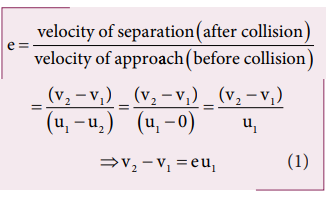Home | | Physics 11th std | Solved Example Problems for Coefficient of restitution (e)

# Solved Example Problems for Coefficient of restitution (e)

Physics : Work, Energy and Power : Coefficient of restitution (e)

Solved Example Problems for Coefficient of restitution (e)

### Example 4.22

Show that the ratio of velocities of equal masses in an inelastic collision when one of the masses is stationary is

v1/v2 = 1-e/1+e

### SolutionFrom the law of conservation of linear momentum,Using the equation (2) for u1 in (1), we getStudy Material, Lecturing Notes, Assignment, Reference, Wiki description explanation, brief detail
11th Physics : UNIT 4 : Work, Energy and Power : Solved Example Problems for Coefficient of restitution (e) |